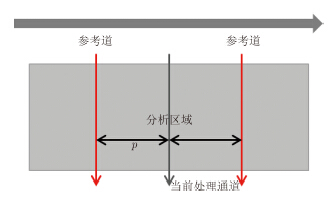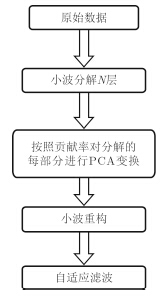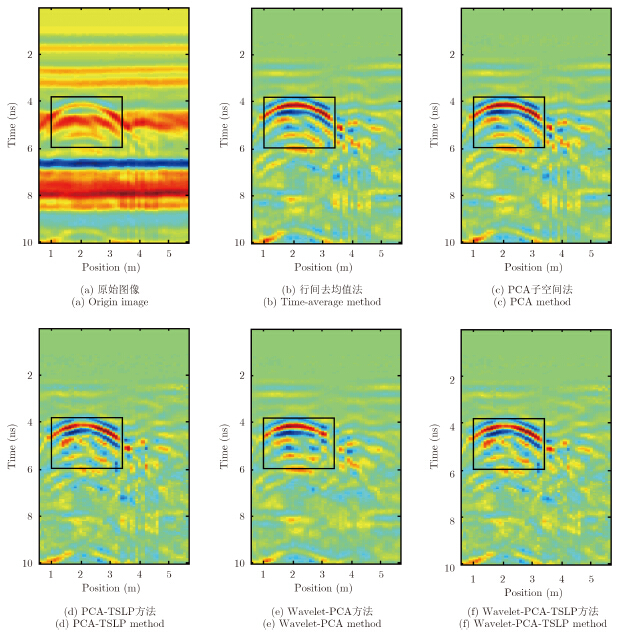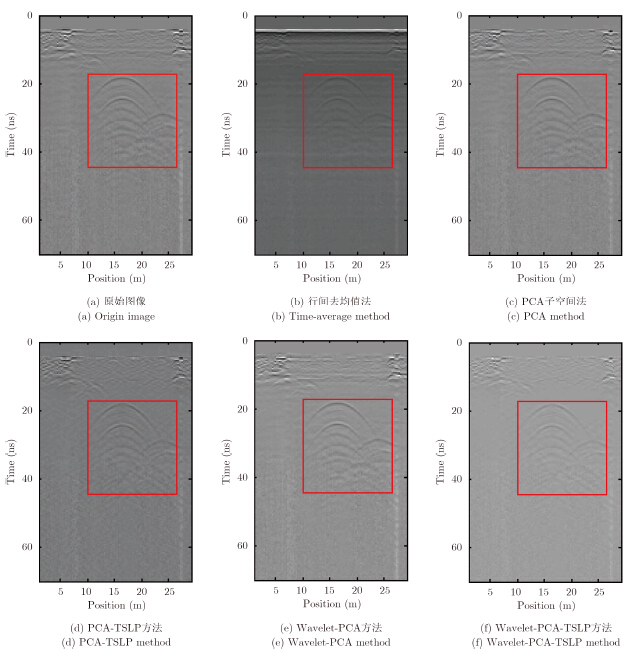﻿ 基于小波变换与主成分分析的探地雷达自适应杂波抑制方法研究
«上一篇文章快速检索 高级检索

 雷达学报2015, Vol. 4 Issue (4): 445–451DOI: 10.12000/JR150130

### 引用本文 [复制中英文]

[复制中文]
Qin Yao, Huang Chun-lin, Lu Min, et al.. Adaptive clutter reduction based on wavelet transform and principal component analysis for ground penetrating radar[J]. Journal of Radars, 2015, 4(4): 445–451. DOI: 10.12000/JR15013.
[复制英文]

### 文章历史,, ,

Adaptive Clutter Reduction Based on Wavelet Transform and Principal Component Analysis for Ground Penetrating Radar,, ,
School of Electronic Science and Engineering, National University of Defense Technology, Changsha 410073, China;
Engineer Academy of People’s Liberation Army, Xuzhou 221000, China
Foundation Items: The National Natural Science Foundation of China (61372160);The Foundation for Doctoral Supervisor of China (20134307110011)
Abstract: Because of the limitations of traditional Principal Component Analysis (PCA) in clutter reduction, an improved PCA subspace method is proposed based on the 2D wavelet transform. Moreover, the combination of the improved subspace method and adaptive filtering ensures the signal fidelity and learning adaptability of adaptive filtering. Then, an adaptive clutter reduction algorithm based on wavelet transform and PCA, as well as adaptive filtering, is proposed. The experimental results suggest that the proposed method improves the signal to clutter ratio and target image definition.
Key words: Ground Penetrating Radar (GPR)     Clutter reduction     Principal Component Analysis (PCA)     Wavelet transform     Adaptive filtering
1 引言

 ${W_{\rm R}} = UD{V^T} = \sum\limits_{i = 1}^P {{u_i}{D_{i,i}}v_i^T}, P = min\{ M,N\}$ (1)

 $l = \sum\limits_{j = 1}^r {{D_{i,i}}} /\sum\limits_{i = 1}^P {{D_{i,i}}}$ (2)

(1) 对原始探地雷达记录的回波数据进行2维小波变换，得到不同分解次数下近似值、水平细节、垂直细节与对角细节信号。小波变换分解次数决定于实际地质资料的复杂度，文中均采用一次小波分解。

(2) 对小波分解得到的不同信号进行主成分分析，取一定贡献率特征值进行重构。

(3) 对经主成分分析处理后的不同信号进行2维小波反变换。 3 自适应杂波抑制图 1 双边线性预测方法示意图 Fig.1 Illustration of two-sided linear prediction approach
 ${x_m} = \left[ {{x_{m - p}}{x_{m + p}}} \right]\left[ \matrix{ \theta \left( 0 \right) \hfill \cr \theta \left( 1 \right) \hfill \cr} \right] = {A_m}\hat \theta$ (3)

 $\hat \theta \left( n \right) = \hat \theta \left( {n - 1} \right) + K\left( n \right)\left( {{x_m}\left( n \right) - A_{_m}^{\rm T}\hat \theta \left( {n - 1} \right)} \right)$ (4)
 $K\left( n \right) = {{{\lambda ^{ - 1}}P\left( {n - 1} \right)A\left( n \right)} \over {1 + {\lambda ^{ - 1}}{A^{\rm T}}\left( n \right)P\left( {n - 1} \right)A\left( n \right)}}$ (5)
 $P\left( n \right) = {\lambda ^{ - 1}}P\left( {n - 1} \right) - {\lambda ^{ - 1}}K\left( n \right)A_{_m}^{\rm T}P\left( {n - 1} \right)$ (6)图 2 Wavelet-PCA-TSLP流程图 Fig.2 Flow of Wavelet-PCA-TSLP algorithm
4 实验结果与分析图 3 第1组数据的实验结果 Fig.3 Results of first B-scan targets after clutter reduction

 $\rm {SCR} = {{\sum\limits_{i = 1}^I {\sum\limits_{i = 1}^J {{T^2}\left( {i,j} \right)/\left( {IJ} \right)} } } \over {\sum\limits_{m = 1}^M {\sum\limits_{n = 1}^N {{W^2}\left( {m,n} \right)/\left( {MN} \right)} } }}$ (7)

 $Q = {{{{\left( {\sum\limits_{i = 1}^I {\sum\limits_{j = 1}^J {{T^2}\left( {i,j} \right)} } } \right)}^2}} \over {\sum\limits_{i = 1}^I {\sum\limits_{j = 1}^J {{T^4}\left( {i,j} \right)} } }}$ (8)表 1 不同方法对实验数据1的运行时间、信杂比与目标图像熵对比结果 Tab. 1 Comparison between results of first B-scan on time cost, SCR and entropy using different approaches图 4 第2组数据的实验结果 Fig.4 Results of second B-scan targets after clutter reduction表 2 不同方法对实验数据2的运行时间、信杂比与目标图像熵对比结果 Tab. 2 Comparison between results of second B-scan on time cost,SCR and entropy using different approaches

  粟毅, 黄春琳, 雷文太. 探地雷达理论与应用[M]. 北京: 科学出版社, 2006: 120–122. Su Yi, Huang Chun-lin, and Lei Wen-tai. Theory and Application of Ground Penetrating Radar[M]. Beijing: Science Press, 2006: 120–122.(2)  吴仁彪, 刘家学, 张蓓. 探地雷达地杂波抑制方法研究进展[J]. 信号处理, 2005, 21(4A): 510–513. Wu Ren-biao, Liu Jia-xue, and Zhang Bei. Survey on GPR ground bounce removal[J]. Signal Processing, 2005, 21(4A): 510–513.(1)  Van der Merwe A and Gupta I J. A novel signal processing technique for clutter reduction in GPR measurements of small, shallow land mines[J]. IEEE Transactions on Geoscience and Remote Sensing, 2000, 38(6): 2627–2637.(1)  Brunzell H. Detection of shallowly buried objects using impulse radar[J]. IEEE Transactions on Geoscience and Remote Sensing, 1999, 37(2): 875–886.(1)  高守传, 粟毅, 黄春琳, 等. 用平均法实现瞬态信号接收中的直达波抑制[J]. 系统工程与电子技术, 2004, 26(1): 21–25. Gao Shou-chuan, Su Yi, Huang Chun-lin, et al.. Using of mean-subtraction method to suppress the direct wave in instantaneous signal receiving[J]. Systems Engineering and Electronics, 2004, 26(1): 21–25.(1)  高倩, 吴仁彪, 米琦. 基于核ICA的探地雷达地杂波抑制[J]. 现代雷达, 2007, 29(3): 23–27. Gao Qian, Wu Ren-biao, and Mi Qi. Kernel ICA for ground bounce removal with GPR[J]. Modern Radar, 2007, 29(3): 23–27.(1)  王萧. 基于KPCA的探地雷达自适应杂波抑制算法研究[J]. 现代电子技术, 2014, 37(11): 31–33. Wang Xiao. Research on KPCA-based adaptive clutter rejection algorithm for GPR[J]. Modern Electronics Technique, 2014, 37(11): 31–33.(1)  申家全, 闫怀志, 胡昌振 . 基于主成分自动选择准则的探地雷达杂波抑制[J]. 电波科学学报, 2010, 25(1): 83–87. Shen Jia-quan, Yan Huai-zhi, and Hu Chang-zhen. Autoselected rule on principal component analysis in ground penetrating radar signal denoising[J]. Chinese Journal of Radio Science, 2010, 25(1): 83–87. (2)  张蓓, 刘家学, 吴仁彪. 探地雷达子空间地杂波抑制方法研究[J]. 信号处理, 2005, 21(4A): 514–517. Zhang Bei, Liu Jia-xue, and Wu Ren-biao. Research on subspace-based GPR ground bounce removel method[J]. Signal Processing, 2005, 21(4A): 514–517.(1)  杨凤娟, 范运岭. 一种改进的滤除探地雷达直耦波的算法[J]. 地球物理学进展, 2013, 28(3): 1617–1625. Yang Feng-juan and Fan Yun-ling. An improved filter algorithm of direct arrive wave for GPR[J]. Progress in Geophysics, 2013, 28(3): 1617–1625.(1)  陈文超, 师振盛, 汪文秉, 等. 小波变换在去除探地雷达信号直达波的应用[J]. 电波科学学报, 2000, 15(3): 352–357. Chen Wen-chao, Shi Zhen-sheng, Wang Wen-bing, et al.. Suppressing the direct wave noise in GPR data using the 2- D continue directional wavelets[J].Chinese Journal of Radio Science, 2000, 15(3): 352–357.(1)  包乾宗, 陈文超, 高静怀, 等. 探地雷达直达波衰减的Curvelet变换方法[J]. 电波科学学报, 2008, 23(3): 449–454. Bao Qian-zong, Chen Wen-chao, Gao Jing-huai, et al.. Direct wave attenuation technique of GPR by curvelet transform[J]. Chinese Journal of Radio Science, 2008, 23(3): 449–454.(1)  李才明, 王良书, 徐鸣洁, 等. 基于小波能谱分析的岩溶区探地雷达目标识别[J]. 地球物理学报, 2006, 49(5): 1499–1504.Li Cai-ming, Wang Liang-shu, Xu Ming-jie, et al.. Objects recognition of ground penetrating radar in karst regions using wavelet energy spectrum analysis[J].Chinese Journal of Geophysics, 2006, 49(5): 1499–1504.(2)  高守传, 黄春琳, 粟毅. 基于RLS横向滤波自适应抵消法的直达波抑制[J]. 信号处理, 2004, 20(6): 566–571. Gao Shou-chuan, Huang Chun-lin, and Su Yi. Direct wave suppression based on adaptive interference canceling method[J]. Signal Processing, 2004, 20(6): 566–571.(1)  Chan Thomas T C, So H C, and Ho K C. Generalized twosided linear prediction approach for land mine detection[J]. Signal Processing, 2008, 88(4): 1053–1060.(1)  高翔, 姬光荣, 王群, 等. 主元子空间自适应双边预测探地雷达杂波抑制[J]. 电波科学学报, 2010, 25(2): 253–258. Gao Xiang, Ji Guang-rong, Wang Qun, et al.. Clutter reduction for landmine detection using adaptive two-sided linear prediction in PCA subspace[J]. Chinese Journal of Radio Science, 2010, 25(2): 253–258.(2)  周琳. 探地雷达成像技术研究[D]. [博士论文], 国防科学技术大学, 2012: 44–45. Zhou Lin. Research on ground penetrating imaging techniques[D]. [Ph.D. dissertation], National University of Defense Technology, 2012: 44–45.(1)  申家全, 闫怀志, 胡昌振. 基于图像熵的探地雷达杂波抑制效果评价[J]. 电波科学学报, 2011, 26(2): 267–271. Shen Jia-quan, Yan Huai-zhi, and Hu Chang-zhen. Evaluation method based on image entropy for clutter suppression effect of ground-penetrating radar[J]. Chinese Journal of Radio Science, 2011, 26(2): 267–271.(1)